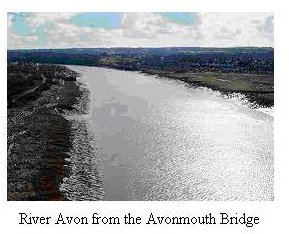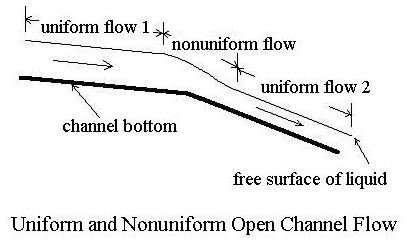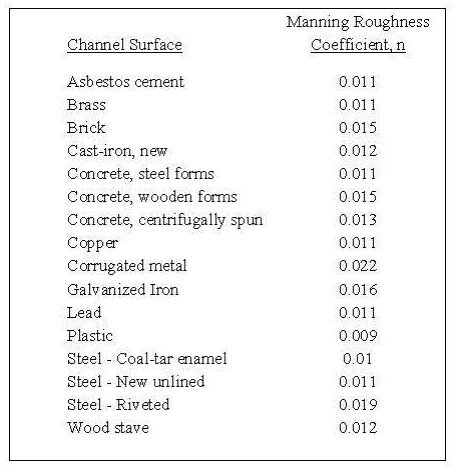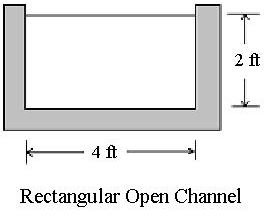# Uniform Open Channel Water Flow Rate Calculation with the Manning Equation

## Introduction

A common use of the Manning Equation is for water flow rate calculation in an open channel. It can also be used to calculate values of other uniform open channel flow parameters such as channel slope, Manning roughness coefficient, or normal depth, when the water flow rate through the open channel is known.An example set of calculations later in this article includes average flow velocity determination and water flow calculation for a given channel and flow depth. The Manning equation applies to open channel flow in natural channels as well as to man-made channels. For example, river discharge can be related to the depth of water flow and river parameters like slope, width and cross-sectional shape.

Image Credit: geograph.org.uk

## Uniform Open Channel FlowUniform open channel flow takes place whenever there's a constant volumetric flow rate of liquid through a section of channel that has a constant bottom slope, constant hydraulic radius (that is constant channel size and shape), and constant channel surface roughness (constant Manning roughness coefficient). Under these conditions, the liquid will flow at a constant depth, often called the normal depth for the given channel and volumetric flow rate. The diagram at the left shows uniform open channel flow in the upstream portion of the channel, followed by a section of non-uniform flow due to a change in channel bottom slope, leading to another stretch of uniform open channel flow. Uniform flow is a necessary condition for the use of the Manning Equation, to be discussed in detail in the next section.

Image Credit: H. Bengtson, Reference #1

## The Manning Equation

The Manning Equation for U.S. units is: Q = (1.49/n)A(R2/3)(S1/2),

and for S.I. units it is: Q = (1.0/n)A(R2/3)(S1/2), Where

Q = volumetric water flow rate passing through the stretch of channel, ft3/sec (m3/s for S.I.)

A = cross-sectional area of flow perpendicular to the flow direction, ft2 (m2 for S.I.)

S = bottom slope of channel, ft/ft (dimensionless),

n = Manning roughness coefficient (empirical constant), dimensionless,

R = hydraulic radius = A/P in ft (m for S.I.) where

A = cross-sectional area of flow as defined above,

P = wetted perimeter of cross-sectional flow area, ft (m for S.I.)

The Manning Equation can be expressed in terms of flow velocity instead of flow rate. Using the equation, V = Q/A as a definition for average flow velocity, the Manning Equation becomes:

V = (1.49/n)(R2/3)(S1/2), with average flow velocity in ft/sec.

In S.I. units this equation becomes: V = (1.0/n)(R2/3)(S1/2), with average velocity in m/s.

Note that the Manning Equation is an empirical, dimensional equation. With the constant equal to 1.49 for U.S. units (or 1.0 for S.I. units), all of the parameters must have the units given above for the chosen system of units.

## The Manning Roughness Coefficient

The Manning roughness coefficient, n, is an experimentally determined constant.Its value depends upon the nature of the channel and its surface. Tables giving values of n for different man-made and natural channel types and surfaces are available in many textbooks, handbooks and on-line. The table above shows Manning roughness coefficient values for severa surfaces commonly used for open channel flow. In general smoother surfaces have lower Manning roughness coefficient values and rougher surfaces have higher Manning roughness coefficient values.

Table Source: Reference #1

## Example Water Flow Rate Calculation for a Rectangular Open Channel

Problem Statement: Consider an open channel of rectangular cross-section, with bottom width of 4ft, containing water flowing 2 ft deep. The bottom slope of the channel is 0.0004 and it is made of concrete with a Manning roughness coefficient of 0.011. What would be the average flow velocity of the water and what would be the volumetric water flow rate?

Solution: The flow velocity and water flow rate calculation can both be made using the Manning Equation in the two forms given in the previous section.

the hydraulic radius is R = A/P = (2)(4)/(4 + 2 + 2) = 1 ft

Substituting values into the velocity form of the Manning equation gives:

V = (1.49/0.011)(12/3)(0.00041/2) = 2.71 ft/sec

The open channel water flow rate can then be calculated:

Q = VA = (2.71 ft/sec)(8 ft2) = 21.7 ft3/sec

Any of the other parameters in the Manning Equation could be calculated if it is the only unknown. For example, the channel bottom slope needed to carry a given flow rate in a channel of given shape and size at a given depth of flow could be calculated. The Manning equation can also be used for experimental determination of the Manning roughness coefficient of a given channel, by measuring all of the other parameters (Q, A, P and S) and calculating the Manning roughness coefficient using the Manning equation.

For downloadable Excel templates that can be used for Manning equation/uniform open channel flow calculations, see the article, "Use of Excel Spreadsheet Formulas for Uniform Open Channel Flow/Manning Equation Calculations."

An article with Excel templates to calculate normal depth for uniform open channel flow is "Calculation of Normal Depth for Open Channel Flow with Excel Formulas."

For information on how to make partially full pipe flow calculations, see: "Use of Excel Spreadsheet Templates for Partially Full Pipe Flow."

## Summary

The Manning equation is useful for a variety of open channel flow calculations involving parameters such as water flow rate, flow velocity, channel slope, channel roughness, water flow depth, and channel size and shape parameters. For a natural channel, river discharge (water flow rate) is often a parameter to be determined.

## References and Image Credits

1. Bengtson, Harlan H., Open Channel Flow I – The Manning Equation and Uniform Flow, an online, continuing education course for PDH credit.

2. U.S. Dept. of the Interior, Bureau of Reclamation, 2001 revised, 1997 third edition, Water Measurement Manual.

3. Chow, V. T., Open Channel Hydraulics, New York: McGraw-Hill, 1959.

## This post is part of the series: Uniform Open Channel Flow and the Manning Equation

The Manning equation is widely used for uniform open channel flow calculations with natural or man made channels. The Manning equation is used to relate parameters like river discharge and water flow velocity to hydraulic radius, and open channel slope, size, shape, and Manning roughness.